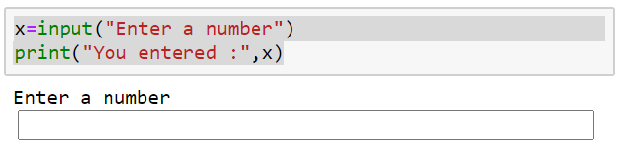# input()

input([prompt]) Accepting user input

prompt: (optional) String ( message ) to be shown to userGetting user input in Python & printing output after converting into integer by using int() function

## Displaying input box to accept user inputs

``````x1=input("Enter first number: ")
x2=input("Enter second number: ")
print("Total :",x1+x2)``````
Output
``````Enter first number: 3
Enter second number: 4
Total : 34``````
Why the output is not equal to 7 with input of 3 and 4 as input numbers ?

As all user inputs are string data type ( by default ) , we have to convert the type by using int()
``````x1=int(input("Enter first number: "))
x2=int(input("Enter second number: "))
print("Total :",x1+x2)``````
Output
``````Enter first number: 3
Enter second number: 4
Total : 7``````

## Float input

Used float() to convert string to float data type.
``````x1=float(input("Interest Rate is : "))
print ("interest rate ",x1)``````
Output
``````Interest Rate is : 5.6
interest rate  5.6``````
``````sum=0
for i in range(3):
x1=int(input('Enter a number'))
sum=sum+x1
print("Sum : ", sum)``````

Subscribe to our YouTube Channel here

## Subscribe

* indicates required
Subscribe to plus2netplus2net.com